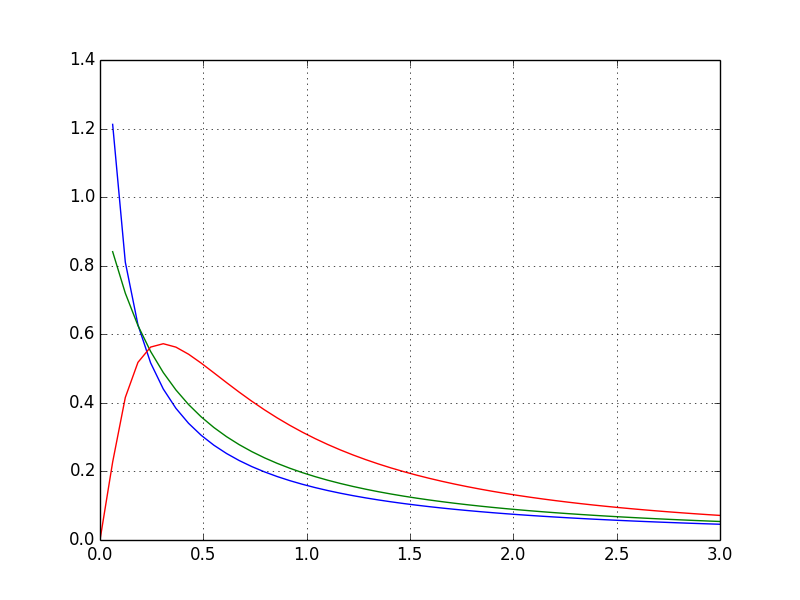[PYTHON] Frequently used subpackages of SciPy

SciPy is a collection of many mathematical algorithms and useful functions that is based on NumPy and is an extension of it. Today, I'm going to explore some of the huge SciPy subpackages that are often used and useful. I will.

The subpackages include: Each of these can be called from scipy import PACKAGE_NAME. (There are other ways to do it)

package Contents
cluster Clustering algorithm
constants Physical constants and mathematical constants
fftpack Fast Fourier transform routine
integrate Integration and ordinary differential equations
interpolate Interpolating and smoothing splines
io Input and output
linalg linear algebra
ndimage N-dimensional processing
odr Total least squares
optimize Optimization and root detection routine
signal Signal processing
sparse Routines associated with sparse matrices
spatial Spatial data structures and algorithms
special Special features
stats Statistical distribution and function
weave C/C++Integration

At my own discretion and prejudice, the most frequently used subpackages are scipy.stats and scipy.linalg. .

Statistical function (scipy.stats)

scipy.stats is a subpackage for statistics. First, there are two general classes that encapsulate continuous random variables and discrete random variables. Based on this, SciPy has classes for over 80 continuous random variables and over 10 discrete random variables. These primarily statistics classes are grouped under scipy.stats.

Common methods for continuous random variables include:

Method Contents
rvs Random variate
pdf Probability density function
cdf Cumulative distribution function
sf Survival function (1-CDF）
ppf Percentage point function (inverse of CDF)
isf Reverse survival function (inverse of SF)
stats Mean, variance, fisherman kurtosis, likelihood
moment Decentralized product ratio

F distribution

It is a familiar F distribution.

from scipy.stats import f #Call the F distribution from stats
def draw_graph(dfn, dfd):
rv = f(dfn, dfd) #Draw an F distribution with the given two arguments
x = np.linspace(0, np.minimum(rv.dist.b, 3))
plt.plot(x, rv.pdf(x))

draw_graph(1, 1)
draw_graph(2, 1)
draw_graph(5, 2)z score

It can be obtained as follows.

x = np.array([61, 74, 55, 85, 68, 72, 64, 80, 82, 59])
print(stats.zscore(x))
#=> [-0.92047832  0.40910147 -1.53413053  1.53413053 -0.20455074  0.20455074
#    -0.61365221  1.02275369  1.22730442 -1.12502906]

Linear algebra (scipy.linalg)

Very fast linear algebra calculations using BLAS and LAPACK ..

All linear algebra routines assume an object that can be transformed into a two-dimensional array. The output of these routines is also basically a two-dimensional array.

x = np.array([[1,2],[3,4]])
linalg.inv(x)
#=>
# array([[-2. ,  1. ],
#        [ 1.5, -0.5]])

Summary

SciPy's subpackages have a huge number of functions and are far from explainable. If you are interested, please read the Online Documents.

If you are not satisfied with the online documents, you can also refer to Free books introduced earlier.